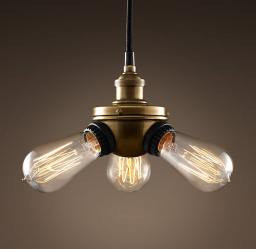# Suppose 6

Suppose the life span of a revolutionary light bulb is normally distributed with a mean life span of 70 thousand hours and a standard deviation of 3 thousand hours. If a light bulb is chosen at random:

a) what is the probability the life span will be within 5 thousand hours of the mean;
b) what must its lifetime be if it is to be categorized in the tallest 5%?

p =  0.9522
t2 =  76000 h

### Step-by-step explanation:Did you find an error or inaccuracy? Feel free to write us. Thank you!

Tips for related online calculators
Looking for help with calculating arithmetic mean?
Looking for a statistical calculator?
Looking for a standard deviation calculator?
Need help calculating sum, simplifying, or multiplying fractions? Try our fraction calculator.
Do you want to convert time units like minutes to seconds?

#### Grade of the word problem:

We encourage you to watch this tutorial video on this math problem: